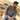# Learn SVG basicsTaimoor Sattar

SVG (Scalable Vector Graphics) is a two-dimension vector-based graphic, ** supports animation and interaction**. SVG can be rendered to any size without loosing quality.

Bitmapped image formats, such as JPEG or PNG, distort as the size increases. In order to display a bitmapped image in a higher resolution, we need a larger matrix and more pixels in the grid.

In HTML, we can write svg as below:

``````<svg width="960" height="500">
</svg>

<!-- or -->

<svg viewBox="0 0 960 500">
</svg>``````

Using the viewBox attribute, we can define the position and dimension.

In `<svg>`, we can use the `<g>` tag to group elements. For example, if we want to apply the certain transformations to the group of SVG elements, we can use the `<g>` tag.

``````<svg viewBox="0 0 63 21" xmlns="http://www.w3.org/2000/svg">
<!-- Using g to inherit presentation attributes -->
<g fill="white" stroke="green" stroke-width="1">
<circle cx="10.5" cy="10.5" r="10"></circle>
<circle cx="31.5" cy="10.5" r="10"></circle>
<circle stroke="blue" cx="52.5" cy="10.5" r="10"></circle>
</g>
</svg>``````

In the CSS, we can change the

``````svg{
background-color: red;
}``````

In the above code, we have defined three circles with a stroke width of 1. First, two circles inherit the property as define in the `<g>` tag. The third (last) circle has the stroke color of blue. To visualize how the above code work, see below:

Rather than a circle, we can also draw more shapes in SVG.

## Basic Shapes in SVG

In SVG, we can draw seven(7) basic shapes as below:

• Path `<path>`
• Rectangle `<rect>`
• Circle `<circle>`
• Ellipse `<ellipse>`
• Line `<line>`
• Polyline `<polyline>`
• Polygon `<polygon>`

### Path in SVG

The `<path>` element is used to define a path. The below command is available to define the path.

• M = moveto
• L = lineto
• H = horizontal lineto
• V = vertical lineto
• C = curveto
• S = smooth curveto
• Q = quadratic Bézier curve
• T = smooth quadratic Bézier curveto
• A = elliptical Arc
• Z = closepath

In HTML, we can define a path as below:

``````<svg height="210" width="400">
<path d="M150 0 L75 200 L225 200 Z" />
</svg>``````

In the above example, the path starts at a position (150,0) with a line to position (75,200) from there, a line to position (225,200), and finally closing the path back to (150,0).

### line in svg

The `<line>` element is an SVG used to create a line connecting two points.

``````<svg viewBox="0 0 100 100">
<line x1="20" y1="80" x2="100" y2="20" stroke="black" />
</svg>``````

### Rectangular in svg

The `<rect>` element is used to create a rectangle and variations of a rectangle shape:

``````<svg width="400" height="180">
<rect x="50" y="20" width="150" height="150"/>
</svg>``````

In CSS, we can style below:

``````rect{
fill:blue;
stroke:pink;
stroke-width:5;
fill-opacity:0.1;
stroke-opacity:0.9
}``````

### Circle in SVG

The `<circle>` SVG element is used to draw a circle, based on the position and radius. In HTML, we can draw svg circle as below:

``````<svg>
<circle cx="10" cy="10" r="10"/>
</svg>``````

### ellipse in svg

The `<ellipse>` SVG element is used to draw an ellipse, based on a center coordinate, and both their x and y radius.

In HTML, we can draw SVG ellipse as below:

``````<svg height="140" width="500">
<ellipse cx="200" cy="80" rx="100" ry="50" />
</svg>``````

### polyline in svg

The element is used to create any shape that consists of only straight lines (that is connected at several points):

``````<svg height="140" width="500">
<polyline points="0 100, 50 70, 60 40, 20 0" />
</svg>``````

### polygon in svg

The element is used to create a graphic that contains at least three sides. Polygons are made of straight lines, and the shape is “closed”.

``````<svg height="200" width="200">
<polygon points="25 100, 120 110, 170 40, 20 0" />
</svg>``````

If you want to draw complex SVG art, you have to be good at math. I prefer to draw SVG on illustration software e.g. Photoshop, Affinity Designer, Gimp, etc. Once you finalize the SVG, you can then export it in SVG format.